## LetsPlayMaths.Com

WELCOME TO THE WORLD OF MATHEMATICS

# Class-8 Square And Square Roots

Introduction to Square

Square Numbers / Perfect Squares

Facts of Perfect Squares

Properties of Perfect Square

Number Patterns of Square Numbers

Square Roots

Methods of finding square roots

Prime factorisation Method

Division Method

Square And Square Roots Test

Square And Square Roots Worksheets

## Introduction to Square

As we know, area of a square is the product of it's two sides i.e. square of sides (side)2. If we consider the side of a square is 'a' unit, then Area of square = (a × a ) square unit = a2 sq. unit. From this we conclude that, square of a number is the product of the number by the same number.

Example 1. 22 = 2 × 2

Example 2. (13)2 = 13 × 13

## Square Numbers / Perfect Squares

Square numbers also known as Perfect square. For example, 9, 16, 25, 36, 49, 64, 81, ... are perfect squares.

Number Square
1 1
2 4
3 9
4 16
5 25
6 36
7 49
8 64
9 81
10 100

## Facts of Perfect Squares

All the natural numbers are not perfect square, but square of natural numbers are perfect squares.

If a natural number is N, and it is expressed as M2. Here M is natural number and N is a perfect square. But all the natural numbers do not form perfect square. From the below examples, we can know whether a given natural number is a perfect square.

Example 1. 25 is a square number because it is expressed by multiplying two same natural numbers of 5.
25 = 5 × 5

Example 2. 18 is not a square number because it can be expressed by multiplying different natural numbers like
18 = 3 × 6 = 2 × 9

A perfect square can always form by multiplying the pairs of equal prime factors.

When pairs of equal prime factors are multiplied with each other they form a perfect square. But when the prime factors are multiplied without pairing, they do not form a perfect square. Let's see some examples.

Example 1. 36 = 2 × 2 × 3 × 3. (Here 36 is perfect square)

Example 2. 108 = 2 × 2 × 3 × 3 × 3. (Here 108 is not a perfect square as 3 cannot paired)

When a smallest natural number is multiplied by a non-perfect square to make it a perfect square.

Example 1. Find the smallest natural number that multiplied by 108 to make it a perfect square.

Solution. Given number is 108. First we must calculate its prime factors by using prime factorisation method. Then arrange the prime factors as the product of pairs of equal prime number and unpaired prime number as shown below.

108 = 2 × 2 × 3 × 3 × 3

We can see, 3 is left unpaired. So, to make 108 a perfect square another 3 is required. Thus 108 is multiplied by 3 to get the perfect square.

Hence 3 is the smallest number.

When a smallest natural number is divided by a non-perfect square to make it perfect square.

Example 1. Find the smallest natural number that will divide 48 to make it a perfect square?

Solution. Here the given number is 48. First we must calculate its prime factors by using prime factorisation method. Then arrange the prime factors as the product of pairs of equal prime number and unpaired prime number as shown below.

48 = 2 × 2 × 2 × 2 × 3

Here number 3 is left unpaired. So to make 48 a perfect square we divide it by 3.

Hence 3 is the smallest number.

## Properties of Perfect Square

The natural number ends with 0, 1, 4, 5, 6, or 9 at its unit's place are not square numbers. But a square number always ends with 0, 1, 4, 5, 6, or 9 at it's unit place. A number having 2,3,7,or 8 at its unit's place is never a square number.

Example 1. Check if 2978 is a perfect square number.

Solution. 2978 is not a perfect square, because it ends with 8 at its unit's place.

Example 2. Check if 76302 is a perfect square number.

Solution. 76302 is not a perfect square, because it ends with 2 at its unit's place.

Example 3. Check if 289 is a perfect square number.

Solution. 289 is a perfect square number, because it ends with 9 at its unit's place.

Example 4. Check if 3253 is a perfect square number.

Solution. 3253 is not a perfect square number, because it ends with 3 at its unit's place.

A number which has odd number of zeros at the end will never be a perfect square. Perfect square always ends with even number of zeros.

Example 1. Check if 90000 is a perfect square number.

Solution. 3002 = 90000
We can notice 4 zeros are present in 90000, hence it is a perfect square.

Example 2. Check if 2500 is a perfect square number.

Solution. 502 = 2500
We can notice 2 zeros are present in 2500, hence it is a perfect square.

Example 3. Check if 400000 is a perfect square number.

Solution. We can notice 5 zeros are present in 400000, hence it is not a perfect square.

Example 4. Check if 2000 is a perfect square number.

Solution. We can notice 3 zeros are present in 2000, hence it is not a perfect square.

Example 5. Check if 40 is a perfect square number.

Solution. We can notice only 1 zero is present in 40, hence it is not a perfect square.

Square of odd natural numbers always get odd natural number.

Example 1. 3792 = 1,43,641 is odd because 379 is an odd natural number.

Example 2. 1372 = 18,769 is odd because 137 is an odd natural number.

Square of even natural numbers is always getting even number.

Example 1. 642 = 4,096 is even because 64 is an even natural number.

Example 2. 322 = 1,024 is even because 32 is an even natural number.

The square unit place number depends upon the number present in unit's place of natural number.

If a number ends with 1 or 9 in the unit's place, then its square unit's place will have 1.
92 = 81, 312 = 961

If a number ends with 0 in the unit's place, then its square ends in 0.
102 = 100

If a number ends with 5 in the unit's place, then its square ends in 5.
252 = 625

If a number ends with 3 or 7 in the unit's place, then its square ends in 9.
132 = 169, 172 = 289

If a number ends with 2 or 8 in the unit's place, then its square ends in 4
122 = 144, 82 = 64

If a number ends with 4 or 6 in the unit's place, then its square ends in 6.
142 = 196, 162 = 256.

## Number Patterns of Square Numbers

1. Non square numbers between two consecutive square numbers
2. Adding consecutive odd numbers to express perfect square number
3. Square of odd number greater than one is expressed by adding two consecutive natural number
4. Square of numbers with digits 5 at unit's place
5. Pythagorean triplet

### Non square numbers between two consecutive square numbers

If we consider two consecutive numbers (p) and (p+1), then there are 2p non square numbers present in between p2 and ( p+1)2. Let's see some examples.

Example 1. How many non-square natural numbers are present between 52 and 62?

Solution. Two perfect square numbers are 52 = 25 and 62 = 36

As per the formula we will be having 10 (2 × 5) non-square natural numbers.

In order to prove this, we have to find out the numbers present between 25 and 36.

And the numbers are 26, 27, 28, 29, 30, 31, 32, 33, 34, and 35.

So, the total count of numbers are 10.

### Adding consecutive odd numbers to express perfect square number

To get perfect square number, consecutive odd natural numbers should be added starting from 1. Let's see some examples.

Example 1. Express 64 as the sum of the numbers of odd number.

Solution. 64 = 1 + 3 + 5 + 7 + 9 + 11 + 13 + 15 = 82

Here we add first 8 odd natural number and get square of 8 i.e. 64.

If a natural number not expressed as a sum of consecutive odd natural numbers starting from 1, then that number is not a perfect square.
In other word, to know a Number is a perfect square, we must subtract all first odd natural numbers (starting from 1) from the given square number untill the result is zero. Let's see some examples.

Example 1. Check if number 65 is perfect square.

Solution. Consecutively subtract 1, 3, 5, 7, ... from number 65 untill we get zero. If the answer comes out to be zero then we can conclude the number to be perfect square.

65 − 1 = 64, 64 − 3 = 61, 61 − 5 = 56, 56 − 7 = 49, 49 − 9 = 40, 40 − 11 = 29, 29 − 13 = 16, 16 − 17 = -1.

Here, we can see the answer turns out to be −1.

So, we can conclude that number 65 is not a perfect square.

Example 2. Check if number 16 is perfect square.

Solution. Consecutively subtract 1, 3, 5, 7, ... from number 16 untill we get zero.

16 − 1 = 15, 15 − 3 = 12, 12 − 5 = 7, 7 − 7 = 0

Here, we can see the answer turns out to be 0.

So, we can conclude that number 16 is a perfect square.

### Square of odd number greater than one is expressed by adding two consecutive natural number

Square of any odd number greater than 1 is expressed by summing of consecutive natural numbers. But sum of any two consecutive integers does not always form perfect square.

There is a formula to calculate first number and second number.
Let's assume perfect square number is n2.
First number is calculated by using formula n2-12
Second number is n2+12

Example 1. Find the two consecutive natural numbers whose summation will be equal to 132.

Solution. First number = 132-12 = 84

Second number = 132+12 = 85

132 = 169 = 84 + 85.

Example 2. Find the two consecutive natural numbers whose summation will be equal to 392.

Solution. First number = 392-12

= (1521-1)2 = 760

Second number = 392+12

= (1521+1)2 = 761

So, 392 = 760 + 761.

### Square of numbers with digits 5 at unit's place

Here is the formula to find out square of numbers having 5 at the unit place.
(m5)2 = m(m + 1)hundreds + 25 = m(m + 1) × 100 + 25
Here m is digits before the units place. Let's see some examples.

Example 1. Find the square of 45.

Solution. As per the above mentioned formual here m is 4.

452 = 4(4+1)hundred + 25

= {(4 × 5) × 100} + 25

= 20 × 100 + 25

= 2000 + 25

= 2025

Example 2.Find the square of 235.

Solution. Here m is 23.

2352 = 23(23+1)hundred + 25

= {(23 × 24) × 100} + 25

= 552 × 100 + 25

= 55200 + 25

= 55225

### Pythagorean triplet

If we consider P, Q and R are three natural numbers and they are following the Pythagorean theory that is P2 + Q2 = R2 then these three natural numbers form Pythagorean triplet.
For any natural number P > 1, then (2P)2 + (P2 − 1)2 = (P2 + 1)2. So here( 2P, P2−1, P2+1 ) are formed Pythagorean triplet.
Let's see some examples.

Example 1. Find Pythagorean triplet, whose smallest number is 16.

Solution. Let us consider 2P = 16.

=> P = 162

=> P = 8

Now we put the value of P in P2−1 = 82−1 = 63

Now we put the value of P in P2 + 1 = 82 + 1 = 65

The Pythagorean triplets are (16, 63, and 65).

Example 2. Find Pythagorean triplet with one number is 18.

Solution. Let us first consider P2 − 1 = 18 , P2 = 18 − 1 = 17. So, here value of P will not a natural number.

Now, we consider P2 + 1 = 18, P2 = 18 + 1 = 19. So, here value of P will not a natural number.

Now, consider 2P = 18, P = 182 = 9. So, now we can put the value of P = 9 in

P2 − 1 = 92 − 1 = 80, and P2 + 1 = 92 + 1 = 82.

Then the collection of numbers (18, 80, 82) is Pythagorean triplet with one number is 18.

## Square Roots

Square root, is a factor of a number, that when multiplied by itself gives the original number.
If M is the square root of N, then M × M = N
The square root of number N is shown as  N .
So,  N  = M.

NOTE - finding the square root is the inverse operation of finding square.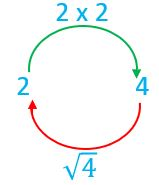## Methods of finding square roots

1. Prime factorisation method
2. Division method

## Prime factorisation Method

To find square root of natural numbers

Step 1. The given number is expressed into product of prime factors.
Step 2. Make the prime numbers in pair of same prime number.
Step 3. Consider one prime number from each pair and multiply them together.
Step 4. The product is known as square root of the given number.
Let's see some examples.

Example 1. Find the square root of 256 by prime factorisation.

Solution. First convert 256 into prime factors by prime factorisation method.

256  =  2×2×2×2×2×2×2×2

=  2×2×2×2×2×2×2×2

= 2×2×2×2 (Taking one prime number from each pair)

So,  256  = 16.

To find square root fraction

First, we should calculate the square root of numerator and then denominator of the fraction.
Square root of fraction = Square root of numerator/Square root of denominator.

Example 1. Find the square root of 196144.

Solution. √(196144) =  196  144

=  (2×2×7×7)  (2×2×2×2×3×3)

= √(22×72)√(22×22×32)

= (2×7)(2×2×3)

= 1412

= 76

To find square root of decimal number

We should first convert it to fraction, then express it to lowest term if needed then calculate square root as method used for fraction above.

Example 1. Find the square root of 2.89.

Solution. First convert the decimal number 2.89 to fraction.

2.89 = 289100

Then calculate square root of 289100

√(289100) =  289  100

=  17×17  10×10

= 1710

= 1.7

Hence square root of 2.89 = 1.7.

To find square root of mixed fraction number

First, we must convert the mixed fraction into improper fraction. Then find out the square root using normal fraction method we dicuseed above.

Example 1. Find the square root of 426125

Solution. First convert mixed fraction into improper fraction number.

426125 = 36125

Now, calculate the square root of 36125

= √(36125)

=  361  25

=  (19×19)  (5×5)

= √(19)2√(5)2

= 195

= 345

Hence, square root of 426125 is 345.

## Division Method

This method is used to calculate square root of large number. It is also called long division method. Let's see some examples to understand the process.

Example 1. Find the square root of 529.

Solution. Let's discuss it stepwise.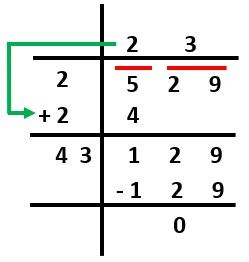• First put bars over the pair of digits from right to left starting from unit's place.
• If number of digits is odd, bar must be put over remaining single digit that is present extreme left.
• Then first consider the first pair of digit or single digit number.
• Here, first digit is 5 now find out greatest number whose square is less than or equal to 5.
• Here, we get that number is 2.Then write 2 in the place of divisor and on the top in the quotient.
• Then subtract 4 from 5 and we get remainder as 1.
• Next pair of digits 29 is brought down and written near to 1 and we get new dividend as 129
• Add divisor with its quotient, here we get 4 and it became a new divisor for new dividend as written above.
• Keep some space right to 4.
• Then find the largest digit to fill that blank and it also become new digit in the quotient.
• The new divisor is multiplied by new digit in the quotient, then product is less than or equal to the dividend.
• So, here 43 multiplied by 3 and we get 129, that means new digit we choose is 3.
• Then subtract 129 from 129 and we get zero as shown above.
• Hence,  529  = 23.

Example 2. Find the square root of 7396.

Solution. Let's discuss it step wise.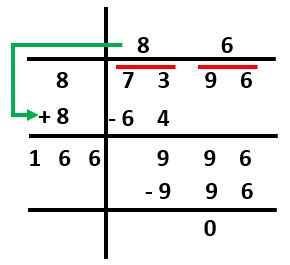• Put bar over the pair of digits from right to left starting from unit's place.
• Then consider the first pair of digits i.e. 73.
• Find out greatest number whose square is less than or equal to 73.
• We get the number as 8. Then write 8 in the place of divisor and in the place of quotient as shown here.
• Subtract 64 from 73, and we get remainder as 9.
• Next pair of digits 96 is brought down and written near to 9 and we get new dividend as 996
• Add the divisor with its quotient, and we get 16 as our new divisor for new dividend 996.
• Keep some space right to 16.
• Then find the largest digit to fill that blank and it also become new digit in the quotient.
• The new divisor is multiplied by new digit in the quotient, then product is less than or equal to the dividend.
• In this case, 166 multiplied with 6 and we get 996. That means, new digit we choose is 6.
• Subtract 996 from 996 and get zero as shown above.
• Hence,  7396  = 86

### Square roots of Decimal by long division method

Following method is used to calculate square root of decimal number. Let's see the below given example.

Example 1. Find the square root of 19.9809.

Solution. Let's discuss it step wise.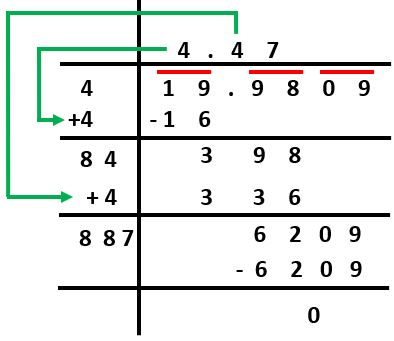• Put bars on the pair of integer present before decimal point from right to left and put bars over the pair of integers from left to right after decimal point.
• First consider the first pair of digits i.e. 19.
• Now find out greatest number whose square is less than or equal to 19. And the number is 4.
• Write 4 in the place of divisor and on the top in the place of quotient.
• Subtract 16 from 19 and we get remainder as 3.
• As next pair of digits come after decimal point, so we should write the decimal point in the quotient.
• Then next pair of digits 98 is brought down and written next to 3 and we get new dividend as 398
• Add divisor with its quotient, we get 8 and it became a new divisor for new dividend as written above.
• Space must be blank right to 8.
• Find the largest digit to fill that blank and it also become new digit in the quotient.
• The new divisor is multiplied by new digit in the quotient, then product is less than or equal to the dividend.
• So, here 84 multiplied with 4 and get 336 that means new digit we choose is 4.
• Subtract 336 from 398, we get 62 as shown above.
• Then next pair of digits 09 is brought down and written next to 62, we get new dividend as 6209.
• Add divisor 84 with its quotient 4, we get 88 and it became a new divisor for the new dividend 6209.
• Space must be blank right to 88.
• Then find the largest digit to fill that blank and it also become new digit in the quotient.
• The new divisor is multiplied by new digit in the quotient, then product is less than or equal to the dividend.
• So, here 887 multiplied by 7 and we get 6209 that means new digit we choose is 7.
• Subtract 6209 from 6209 and we get zero as shown above.
• Hence,  19.9809  = 4.47

### Square root of a fraction using long division method

In this case, we should first convert the fraction into decimal form and then find out the square root of the decimal number using the method described above.

Example 1. Find the square root of fraction 358 correct to 2 decimal places.

Solution 358 = 298 = 3.625

Now we will calculate the square root of 3.625 by long division method as explained above, then we get  3.625  = 1.903... = 1.90 (correct to 2 decimal)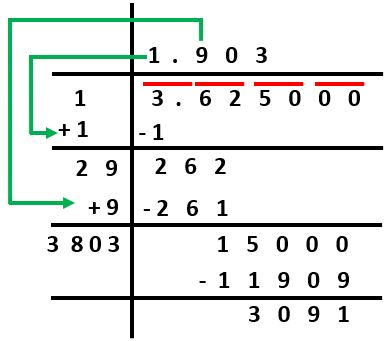## Class-8 Square And Square Roots Test

Square And Square Roots - 1

Square And Square Roots - 2

## Class-8 Square And Square Roots Worksheets

Square And Square Roots Worksheet - 1

Square And Square Roots Worksheet - 2

Square And Square Roots Worksheet - 3